## is it possible to keep GAMMA(1,x) as is and not r...

I am reading some expressions from file.

In the file, it is written as GAMMA(-1,x). When read into Maple using the read file command, it shows as  1/x*Ei(2,x)

I know these are the same mathematically. But I am translating these expressions to sagemath. in sagemath, Ei only accepts one argument.

Now the translator sees Ei in the input, then it keeps it as Ei as it does not know it was the GAMMA with two arguments,  which gives an error when used by sagemath since sagemath only has the one argument version of Ei.

If Maple would keep GAMMA(-1,x) as is, then the translator will just translate it to gamma(-1,x) which works in sage.

To keep things simple, I was wondering if there an option to tell Maple to keep the input as GAMMA(-1,x) and not rewrite to Ei?

Otherwise I would have to now parse each Ei to see if it is the two argument version or the one argument version, and use gamma  for the 2 argument version to keep sage happy which will complicate things for me.

expr:=GAMMA(-1,x)

Ei(2, x)/x

Maple 2022.1

I am trying to rotate a region about the y-axis. However, entering axis=vertical rotates it about the z-axis. I have also tried interchanging the x and y values. However, it produces the same output. The left image is what I'd like to rotate about the y-axis. The code below is for the right image. How would I revolve this region about the y-axis? Please help.

VolumeOfRevolution(2*x^2 + 1, x = 0 .. 1, labels = [x, y, z], output = plot, axis = vertical, orientation = [270, 0, 90])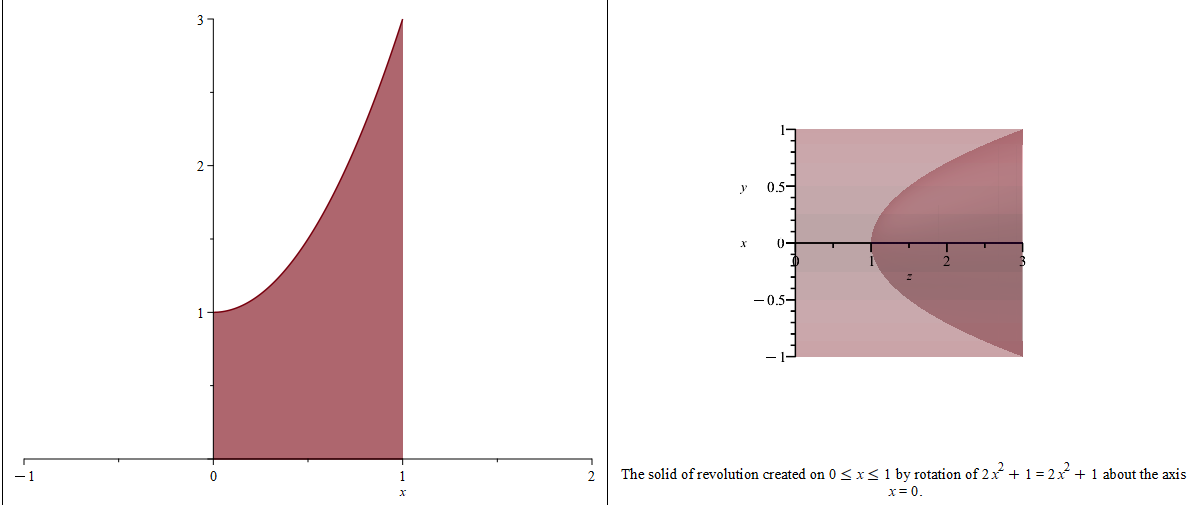## Getting error too many levels of recursion over th...

 >>(1)
 >(2)
 >(3)
 >(4)
 >(5)
 >(6)
 >>>Error, invalid function arguments>Here psi is a general wave function from schrodinger wave equation.

## Can Maple's functions be packaged as executable fi...

The problem comes from the link https://www.mapleprimes.com/questions/234398-Convert-Maple-Code-To-Python-#comment287424.

We know that when we compile a C or C ++ function, it generates an executable file.  Then we are free from source code.  For example. the function below returns a square matrix A where    "A[i, j]" is the distance from vertex i to vertex j in the graph G. My computer system is Windows.

// C Program for Floyd Warshall Algorithm
#include <stdio.h>

// Number of vertices in the graph
#define V 4

/* Define Infinite as a large enough
value. This value will be used
for vertices not connected to each other */
#define INF 99999

// A function to print the solution matrix
void printSolution(int dist[][V]);

// Solves the all-pairs shortest path
// problem using Floyd Warshall algorithm
void floydWarshall (int graph[][V]) {
/* dist[][] will be the output matrix
that will finally have the shortest
distances between every pair of vertices */
int dist[V][V], i, j, k;

/* Initialize the solution matrix
same as input graph matrix. Or
we can say the initial values of
shortest distances are based
on shortest paths considering no
intermediate vertex. */
for (i = 0; i < V; i++)
for (j = 0; j < V; j++)
dist[i][j] = graph[i][j];

/* Add all vertices one by one to
the set of intermediate vertices.
---> Before start of an iteration, we
have shortest distances between all
pairs of vertices such that the shortest
distances consider only the
vertices in set {0, 1, 2, .. k-1} as
intermediate vertices.
----> After the end of an iteration,
vertex no. k is added to the set of
intermediate vertices and the set
becomes {0, 1, 2, .. k} */
for (k = 0; k < V; k++) {
// Pick all vertices as source one by one
for (i = 0; i < V; i++) {
// Pick all vertices as destination for the
// above picked source
for (j = 0; j < V; j++) {
// If vertex k is on the shortest path from
// i to j, then update the value of dist[i][j]
if (dist[i][k] + dist[k][j] < dist[i][j])
dist[i][j] = dist[i][k] + dist[k][j];
}
}
}

// Print the shortest distance matrix
printSolution(dist);
}

/* A utility function to print solution */
void printSolution(int dist[][V]) {
printf ("The following matrix shows the shortest distances"
" between every pair of vertices \n");
for (int i = 0; i < V; i++) {
for (int j = 0; j < V; j++) {
if (dist[i][j] == INF)
printf("%7s", "INF");
else
printf ("%7d", dist[i][j]);
}
printf("\n");
}
}

// driver program to test above function
int main() {
/* Let us create the following weighted graph
10
(0)------->(3)
|         /|\
5 |          |
|          | 1
\|/         |
(1)------->(2)
3           */
int graph[V][V] = { {0,   5,  INF, 10},
{INF, 0,   3, INF},
{INF, INF, 0,   1},
{INF, INF, INF, 0}
};

// Print the solution
floydWarshall(graph);
return 0;
}

The above functions will be packaged as the disall.exe , and then we will move them anywhere in my computer and run it in Powershell.  We don't have to deal with the source code unless we want to change it.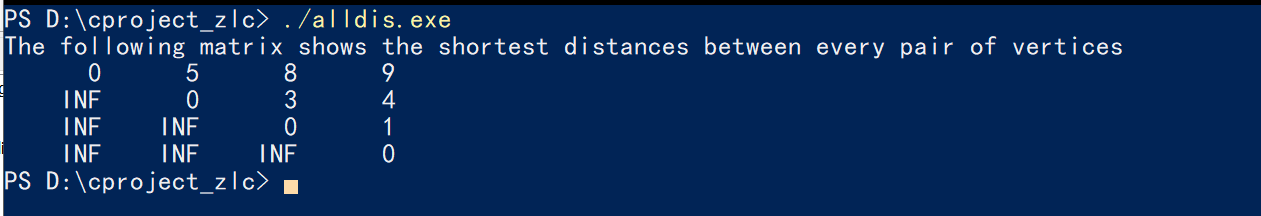I mean can Maple do something like that?

with(GraphTheory);
G := Graph([1, 2, 3, 4, 5], {{1, 2}, {1, 3}, {1, 4}, {1, 5}, {2, 3}, {2, 5}, {3, 4}, {4, 5}});
AllPairsDistance(G);


For exmaple, can I package the above code snippet into an exe file?

## How can I extract the Chebyshev coefficients of th...

Dear All,
I want to extract the coefficients of Chebyshev of an arbitrary function, for example, exp(x). I know that we can use the following command to make a Chebyshev series expansion of exp(x):
chebyshev(exp(x),x);
the above returns the sum of nth Chebyshev polynomials multiplied by Chebyshev coefficients as the following:
1.26606587775201*T(0, x) + 1.13031820798497*T(1, x) + 0.271495339534077*T(2, x) + 0.0443368498486638*T(3, x) + 0.00547424044209371*T(4, x) + 0.000542926311913993*T(5, x) + 0.0000449773229542760*T(6, x) + 3.19843646244580*10^(-6)*T(7, x) + 1.99212480641582*10^(-7)*T(8, x) + 1.10367717095000*10^(-8)*T(9, x) + 5.50589697979079*10^(-10)*T(10, x)

I like to take the coefficients 1.266,1.1303, 0.2714, 0.04433, and so on. How can I do it?
Thanks

## How do I select rows of an array?...

Hi,

I am just starting with Maple after using Mathcad for nearly 30 years. I want to recreate something I have used a lot in Mathcad: select certain rows in array based on a specified criterion. For example, I want to save just the rows that have a certain value in a certain column and save the new array for use later. I am slowly learning how to do it Maple--for instance, I learned that I need to change the variable printlevel if I want to get the output from nested loops.

I currently have the following code:

k := 1;

rows := RowDimension(M);

for i to rows do

if M[i, 3] = "A" then

row(out, k) := row(M, i);

k := k + 1;

end if;

end do;
out;

This doesn't seem to work, though. When I try to display out, I just get the name out instead a matrix of values.

I would appreciate it if someone could give me some idea of what I am doing wrong (with Maple, that is).

Thanks,

John

## How to enter the fluid convective term using the P...

Dear all,

I'm trying to enter an equation (Navier-Stokes) in Maple using the Physics[Vectors] package. I am having trouble with the term

$(u\cdot \nabla) u$

but Maple returns an error when entering the commands :

( u_(t,x,y,z) . %Nabla) u_(t,x,y,z)

I tried other combinations of this without success, like :

( u_(t,x,y,z) . %Nabla)(u_(t,x,y,z))

( u_(t,x,y,z) %. %Nabla) u_(t,x,y,z)

( u_(t,x,y,z) %. %Nabla) (u_(t,x,y,z))

Could you please give me some help with this?

## Fraction of formula too long with typesetting leve...

Hello,
I have a small problem with the display of fraction and x_dot at the same time.

This the exemple :

with(Typesetting);
compactdisplay = flase;
Settings(typesetprime = false);
Settings(typesetdot = true);

diff(1/2*LongExpression*x(t), t);

The Results

when I choose the typesetting level : Extended. I got this result :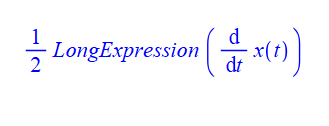And when I choose the typesetting level : Maple Standrad. I got this result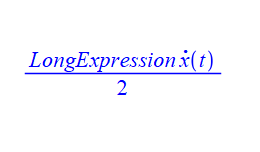What I  want to have is mix of both, it mean like this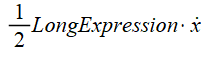## issue while Running Maple...

I have downloaded Maple and install the trial version , i entered the Purchase code  and every thing was done, I did not get any errors , however, while i am trying to open Maple, when I open the software , I see this window which i have uploaded below , and when i click on Active i eneter my purchase code again , and it says it has acitivated and then when i click ok , it exit , what is the issue ?

can you help me ?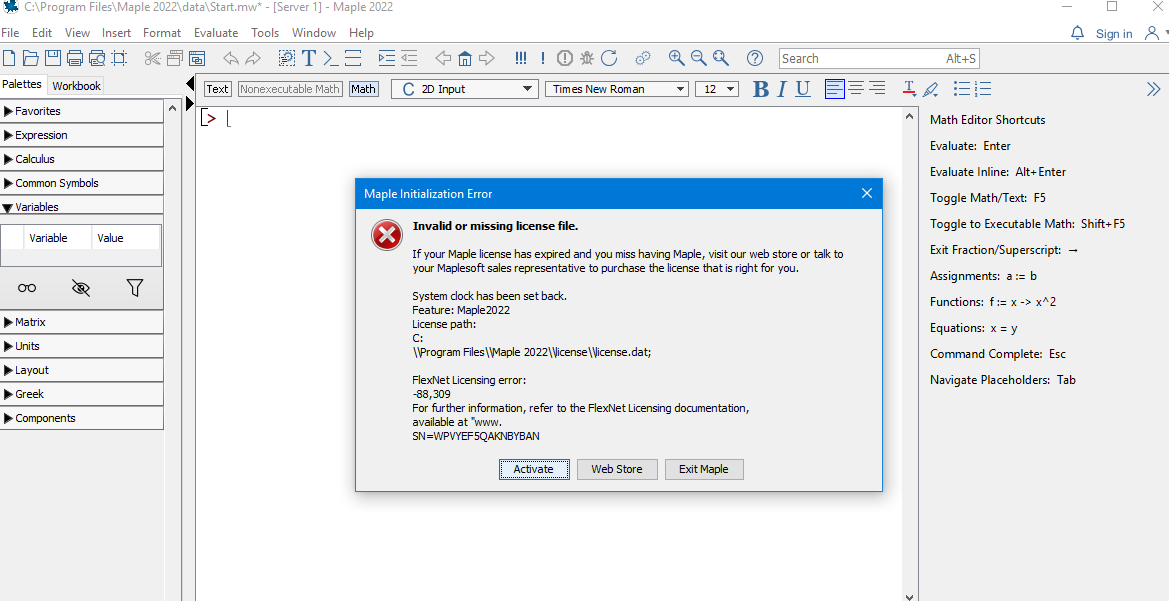## is it possible to have inner module inside object ...

This is the problem: My object is getting large with many private methods.

In non-OOP setup, I could make different modules A,B,C and put relevent methods inside each module.

Now I find I can not do this inside the object. All methods have to be flat and at same level. So I lose the benefit of using different name space for different methods.

It will be easier to explain with a simple example. Lets say I have this now

restart;

A:=module()
option object;
local name::string:="";

export ModuleCopy::static:= proc( self, proto, name::string, $) self:-name := name; end proc; export process_1::static:=proc(_self,$)
_self:-process_2();
end proc;

local process_2::static:=proc(_self,$) print("in process_2 ",_self:-name); end proc; end module;  The above works by having all methods at same level. I can now do o:=Object(A,"me"); o:-process_1() "in process_2 ", "me" But now as the number of private methods increases I want to put a name space around collection of these for better orgnization, but keep all methods logically as part of the object as before. I change the above to be restart; A:=module() option object; local name::string:=""; export ModuleCopy::static:= proc( self, proto, name::string,$)
self:-name := name;
end proc;

export process_1::static:=proc(_self,$) o:-B:-process_2(); end proc; #method process_2 is now inside a module. local B::static:=module() export process_2::static:=proc(_self,$)
print("in B:-process_2 ",_self:-name);
end proc;
end module;

end module;


Now

o:=Object(A,"me");
o:-process_1()


Gives an error

Error, (in unknown) invalid input: process_2 uses a 1st argument, _self, which is missing

I tried many different combinations, but nothing works.

So right now I have all methods flat. All at same level. But I do not like this setup. Since I lose the nice modular orgnization I had with using different modules with different methods inside different modules.

Is there a way to have a module inside an object and call its methods from inside the object as if these method were part of the object private method with only differece is adding the module name in between?

So instead of _self:-foo()  I just change it to  _self:-B:-foo() and have both behave the same way?

In C++, one can use what is called a friend class to do this.

## How do I evaluate double integral with sybolic lim...

int(int(x^(alpha + 1)*y^(beta + 1)*exp(-(x^2 + y^2)/(4*Pi))/sqrt(x^2 + y^2), x = -R .. R), y = -R..R) assuming x>0, y>0, R>0;

I am trying to evaluate the above symbolic integral but Maple returns the same input in Symbolic form without evaluating it at all. Is there something wrong with my approach?## why calling deep call on object makes it no longer...

This is another bizzar behaviour of objects. I created a basic object of type person.  Noticed that after issuing the call copy(object,deep) and not doing anything at all with the result. Just made the call only, then I am not longer able to create new objects of this class. I had to restart the session to be able to create new objects again.

Why?  This seems like something went messy with memory layout. I put a print message in the constructor, and see that this message no longer show up  after the call copy(object,deep) even though this call was not used for any purpose as you see, but to only to see its effect on the session.

Any explanation why one can no longer create new objects after doing this?  Here is the workseet

 > interface(version)> restart;
 > person:=module()     option object;     export name::string:="";       export ModuleCopy::static:=proc(_self,proto, name::string, $) print("Enter constructor of person"); _self:-name := name; end proc; end module;> p1:=Object(person,"me"); p1:-name; do_not_care := copy(p1,deep);> p2:=Object(person,"me"); #WHy constructor no long called? p2:-name; #this no longer work.> p3:=Object(person,"me"); #WHy constructor no long called? p3:-name; #this no longer work.> restart; #had to call restart to fix things.  > person:=module() option object; export name::string:=""; export ModuleCopy::static:=proc(_self,proto, name::string,$)       print("Enter constructor of person");       _self:-name := name;     end proc; end module;> p4:=Object(person,"me");  #now it works again p4:-name;>

## How to remove the mosaic of vertices?...

I  generated some graphs via maple and would like to put them in my paper. So I am going to convert the following worksheet to pdf.

with(GraphTheory):
Graphs:=[NonIsomorphicGraphs(6,8,output=graphs,outputform = graph)]:
num_g:=nops(Graphs):
num:=ceil((num_g)/5.):
M1:=Matrix (num,5,(i,j)->if((i-1)*5+j<=num_g, DrawGraph(Graphs[(i-1)*5+j],size=[250,250] ,overrideoptions ,showlabels=false,style=planar, stylesheet =  [
vertexcolor     = orange
,vertexfontcolor = black
,vertexborder    = false
,edgethickness   = 0.6
,edgecolor       = MidnightBlue
,vertexshape     =  "circle"
,vertexfont      = [Arial, 4],
vertexthickness=5], caption = cat(H__,5*(i-1)+j),captionfont=["ROMAN",7]),plot(x = 0 .. 1, axes = none))):
DocumentTools:-Tabulate (M1[1..5,.. ],widthmode=percentage ,width=80 , exterior =all) :


But there was a problem with the exported pdf. There was some mosaic stuff at the vertices of all those graphs. It was strange. (I want to reduce the size of vertices of the graphs in order not to look crowded.)Only when I insert the option vertexpadding and set a large enough size  of vertex (for this example, we set vertexpadding=7),  it won't go wrong. However, in fact, we often need make vertice‘s size smaller , especially when there are more vertices.